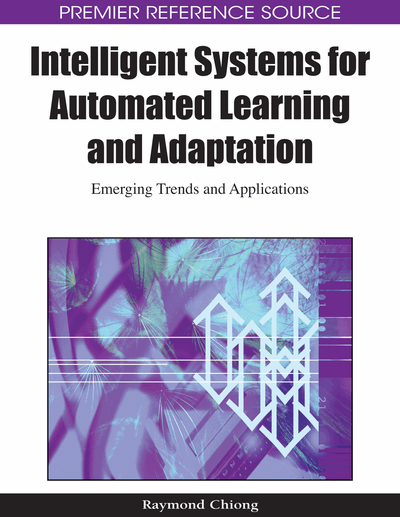# Efficient Training Algorithm for Neuro-Fuzzy Network and its Application to Nonlinear Sensor Characteristic Linearization

Ajoy K. Palit (University of Bremen, Germany) and Walter Anheier (University of Bremen, Germany)
DOI: 10.4018/978-1-60566-798-0.ch004
Available
\$37.50
No Current Special Offers

## Abstract

An ideal linear sensor is one for which input and output values are always proportional. Typical sensors are, in general, highly nonlinear or seldom sufficiently linear enough to be useful over a wide range or span of interest. Due to the requirement of tedious effort in designing sensor circuits with sufficient linearity for some applications, the word nonlinearity has acquired a pejorative connotation. Hence, a computationally intelligent tool for extending the linear range of an arbitrary sensor is proposed. The linearization technique is carried out by a very efficiently trained neuro-fuzzy hybrid network which compensates for the sensor’s nonlinear characteristic. The training algorithm is very efficient in the sense that it can bring the performance index of the network, such as the sum squared error (SSE), down to the desired error goal much faster than any first order training algorithm. Linearization of a negative temperature coefficient thermistor sensor with an exponentially decaying characteristic function is used as an application example, which demonstrates the efficacy of the procedure. The proposed linearization technique is also applicable for any nonlinear sensor (such as J-type thermocouple or pH sensor), whose output is a monotonically increasing/decreasing function.
Chapter Preview
Top

## Introduction

Within the artificial intelligence society, the term computational intelligence is largely understood as a collection of intelligent computational methodologies such as fuzzy-logic-based computing, neuro-computing, and evolutionary computation that help in solving complex computational problems in science and technology which are not solvable or at least not easily solvable by using the conventional mathematical methods (Palit and Popovic, 2005). In the 1990s a growing number of publications on the successful combination of intelligent computational technologies - neural, fuzzy and evolutionary computation - have been reported. Since then hybrid systems, such as neuro-fuzzy networks, have been studied intensively due to their several advantages over the individual constituents. For instance, neural networks have been combined with fuzzy logic resulting in neuro-fuzzy or fuzzy-neural systems in which:

• Neural networks tune the parameters of the fuzzy logic systems, which are used in developing the adaptive fuzzy controllers as implemented in the Adaptive Network-Based Fuzzy Inference System (ANFIS) proposed by Jang (1993).

• Fuzzy logic systems monitor the performance of the neural network and adapt its parameters optimally, for instance, in order to achieve the nonlinear mapping and/or the function approximation to any desired accuracy (Wang and Mendel, 1992a).

• Fuzzy logic is used to control the learning rate of neural networks to avoid the creeping phenomenon in the network while approaching the solution minimum (Arabshahi et al., 1992).

In addition to many applications and improvements, several theoretical results such as universal function approximation capability of neuro-fuzzy networks have also been obtained (Wang, 1994; Wang and Mendel, 1992b). In this chapter, the same feature (universal approximation capability) of the hybrid networks has been exploited for the linearization of highly nonlinear sensor characteristics.

Usually, some particular types of well defined sensor nonlinearities (exponentially rising or exponentially decaying) are compensated by hardware techniques (i.e. by using logarithmic converter) as reported by Patranabis et al. (1988). However, the hardware based linearization procedure lacks the portability i.e. the same procedure cannot be applied so easily to other categories of sensor nonlinearities. In contrast, the linearization technique based on computationally intelligent technology (software based linearization) can very easily be applied to almost all types of sensor nonlinearities. A similar approach but with artificial neural networks has already been reported by Medrano-Marques and Martin-Del-Brio (2001).

However, it is to be noted that the success of software based linearization process based on neural networks and/or neuro-fuzzy networks highly depends on the optimal tuning of (fuzzy) system parameters. Therefore, in this chapter, a training algorithm based on Levenberg-Marquardt approach has been presented that can be applied to fine-tune the free parameters of the hybrid neuro-fuzzy network very efficiently. The training algorithm is very efficient in the sense that it can bring the performance index (sum squared error value) of the network down to the desired error goal much faster than any first order training algorithm (backpropagation algorithm). Thereafter, as an application example, linearization of a negative temperature co-efficient (NTC) type of thermistor sensor has been considered.

The rest of the chapter is organized as follows. The next section presents the structure of the selected neuro-fuzzy network and its training algorithm. Thereafter, the sensor characteristic linearization has been presented as an application example. In the same section, the linearization technique of an arbitrarily chosen nonlinear sensor and the experimental simulations are presented. Finally, the chapter concludes with a brief discussion.

## Complete Chapter List

Search this Book:
Reset